Courses

# Electric Potential Energy of Charged Particles JEE Notes | EduRev

## JEE : Electric Potential Energy of Charged Particles JEE Notes | EduRev

The document Electric Potential Energy of Charged Particles JEE Notes | EduRev is a part of the JEE Course Physics For JEE.
All you need of JEE at this link: JEE

Potential Energy of a System of two Charged Particles

Figure shows two + ve charges q1 and q2 separated by a distance r. The electrostatic interaction energy of this system can be expressed as work done in bringing charge q2 from infinity to the given separation from q1.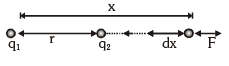It can be calculated as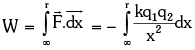[ – ve sign shows that x is decreasing]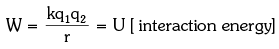If the two charges are of opposite signs, then potential energy will be negative as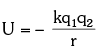Potential Energy for a System of Charged Particles

When more than two charged particles are there in a system, the interaction energy can be given as the sum of interaction energies of all the different possible pairs of particles. For example if a system of three particles having charges q1, q2 and q3 is given as shown in figure.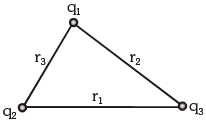The total interaction energy of this system can be given as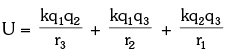Offer running on EduRev: Apply code STAYHOME200 to get INR 200 off on our premium plan EduRev Infinity!

## Physics For JEE

187 videos|516 docs|263 tests

,

,

,

,

,

,

,

,

,

,

,

,

,

,

,

,

,

,

,

,

,

;